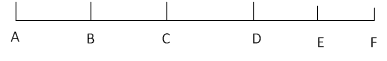### Sample Problem

How many line segments are in the picture?

segments#### Solution

There are five unit segments and six points. We see that there are also four 2-unit segments, three 3-unit segments, two 4-unit segments, and one 5-unit segment. This is a total of 15 line segments.

We can also calculate this using the six points. There are six ways to choose any point and five ways to choose another point. After dividing by two to account for overcounting, we get 6 × 5 ÷ 2 = 15.

Notice that as the number of unit segments increases, it becomes easier to count using the points rather than to count every segment.